﻿ Implementation and Performance Evaluation of OFDM System in Diverse Transmission Channel Using Simulink

Implementation and Performance Evaluation of OFDM System in Diverse Transmission Channel Using Simul...

Arun Agarwal, Kabita Agarwal

American Journal of Electrical and Electronic Engineering

Implementation and Performance Evaluation of OFDM System in Diverse Transmission Channel Using Simulink

Arun Agarwal1,, Kabita Agarwal2

1Department of Electronics and Communication Engineering, Institute of Technical Education & Research, Siksha ‘O’ Anusandhan University, Khandagiri Square, Bhubaneswar, Odisha, India

2Department of Electronics and Telecommunication Engineering, CV Raman College of Engineering, Bhubaneswar, Odisha, India

Abstract

Orthogonal Frequency Division Multiplexing (OFDM) has become a popular modulation method in high data rate wireless communications. It is a key modulation scheme that is especially suited for high-data-rate transmission in delay-dispersive environments because of its robustness to Inter-Symbol Interference (ISI) and multipath fading environment. It converts a high-rate data stream into a number of low-rate streams that are transmitted over parallel, narrowband channels which needs little or no equalization. OFDM also provides efficient spectrum utilization by use of single frequency networks (SFNs). Recently, OFDM has become the standard for physical layer implementation of various digital audio and video wireless communications such as DAB/DVB, wireless local access networks (WLANs) and wireless metropolitan area networks (WMANs). Moreover OFDM is a potential candidate for fourth-generation (4G) mobile wireless systems and beyond. In this paper, through Simulink implementation, we present a bit error rate (BER) performance analysis of OFDM system in diverse transmission channels. The results show that OFDM is well suited for high speed data transmission in mobile environment and channel coding combined with interleaving is mandatory for any wireless high speed transmission.

• Arun Agarwal, Kabita Agarwal. Implementation and Performance Evaluation of OFDM System in Diverse Transmission Channel Using Simulink. American Journal of Electrical and Electronic Engineering. Vol. 3, No. 5, 2015, pp 117-123. http://pubs.sciepub.com/ajeee/3/5/2
• Agarwal, Arun, and Kabita Agarwal. "Implementation and Performance Evaluation of OFDM System in Diverse Transmission Channel Using Simulink." American Journal of Electrical and Electronic Engineering 3.5 (2015): 117-123.
• Agarwal, A. , & Agarwal, K. (2015). Implementation and Performance Evaluation of OFDM System in Diverse Transmission Channel Using Simulink. American Journal of Electrical and Electronic Engineering, 3(5), 117-123.
• Agarwal, Arun, and Kabita Agarwal. "Implementation and Performance Evaluation of OFDM System in Diverse Transmission Channel Using Simulink." American Journal of Electrical and Electronic Engineering 3, no. 5 (2015): 117-123.

 Import into BibTeX Import into EndNote Import into RefMan Import into RefWorks

123
Prev Next

1. Introduction

The concept of OFDM system was first introduced in mid-1960s [Chang1966]. Then Cimini in 1985 evaluated the performance of OFDM for wireless communications. After that in early 1990’s with the development in hardware digital Signal processing made OFDM a realistic option for wireless systems . Recently, OFDM emerged as a key candidate for the high-data-rate applications. Currently, OFDM is used for Digital Audio Broadcasting (DAB), Digital Video Broadcasting (DVB), and Wireless Local Area Networks (LANs) (IEEE802.11a, IEEE802.11g). It will also be used in fourth-Generation (4G) cellular systems & beyond, including Third Generation Partnership Project-Long-Term Evolution (3GPP-LTE) and WiMAX.

OFDM is essentially a discrete implementation of multicarrier modulation, which divides the transmitted bit stream into many different sub streams and sends them over many different sub channels. Typically, the sub channels are orthogonal and the numbers of sub channels are chosen such that each sub channel has a bandwidth much less than the coherence bandwidth of the channel. Thus, inter symbol interference (ISI) on each sub channel is very small. For this reason, OFDM is widely used in many high data rate wireless systems.

In this paper we designed and implemented an OFDM system in SIMULINK and BER performance was evaluated for different transmission channels. In this paper we developed an OFDM transmission system based on IEEE 802.11a standard [4, 5]. The design consists of fundamental signal processing blocks such as QPSK/QAM symbol mapping, convolutional encoder (FEC), interleaving and OFDM signal generator (IFFT) in the transmitter side and in the receiver corresponding inverse operations is carried out. A frame based

Processing is used in this work. Bit error rate (BER) has been considered as the performance index in all analysis.

Following this introduction the remaining part of the paper is Organized as follows. Section 2 provides brief overview of the OFDM system. This section explains the concept and introduces the OFDM system standard. In Section 3, the details of the modelling and simulation of the system using MATLAB is presented. Then, simulation results have been discussed in Section 4. Finally, Section 5 provides the conclusions.

2. The Ofdm System

OFDM is derived from the fact that the high serial bit stream data is transmitted over large (parallel) number sub-carriers (obtained by dividing the available bandwidth), each of a different frequency and these carriers are orthogonal to each other. OFDM converts frequency selective fading channel into N flat fading channels, where N is the number of sub-carriers. Othogonality is maintained by keeping the carrier spacing multiple of 1/Ts by using Fourier transform methods, where Ts is the symbol duration. Since channel coding is applied prior to OFDM symbol generation which accounts for the term coded ‘in COFDM. Orthogonality between sub-carriers is maintained if sinusoids have integer number of cycles in Ts given by (1) and (2) below:

Continuous Time: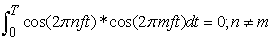(1)

Discrete Time: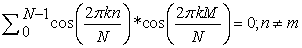(2)

Frequency Division Multiplexing (FDM) divides the channel bandwidth into sub channels and transmits multiple relatively low rate signals by carrying each signal on a separate carrier frequency. To ensure that the signal of one sub channel did not overlap with the signal from an adjacent one, some guard band was necessary which is an obvious loss of spectrum and hence bandwidth. But since carriers are Orthogonal to each other in OFDM therefore it offers bandwidth efficiency as no guard band is required as shown in Figure 1 below.Download asVeiw figureFigures index
Figure 1. Bandwidth conservation (a) FDM (b) OFDM 

Figure 2 below presents the block diagram of an OFDM transceiver. First, the random data bits are mapped to data symbols using digital modulation scheme such as BPSK, QPSK or QAM. Then the serial data stream is converted into a fixed number of parallel data stream. Then, after adding pilot symbols, which are used for channel estimation or synchronization, IFFT operation is performed on each block of symbol which is the OFDM signal. Then an appropriate length of Cyclic prefix or guard interval is appended to the OFDM signal, whose length is chosen to be larger than the maximum delay spread of the channel such that ISI is taken care. After this the OFDM blocks are converted back to serial data, which is converted to analog domain using digital-to-analog converter (DAC) and finally with RF up-conversion sent out to the wireless channel. We assume perfect timing and carrier frequency synchronization at the receiver. Thus, the received signals after down conversion & analog-to-digital conversion (ADC) is transformed to parallel data and then after the cyclic prefix removal, FFT operation is done on each data block. After demodulation, the transmitted original bit stream is recovered. In broadband time-varying multipath fading channels, OFDM has some of its own challenging issues, such as peak-to-average-power ratio (PAPR) and carrier frequency offset (CFO). However, much more unique merits of OFDM make it widely used in wireless applications and standards. The important blocks are described in brief as follows:

A. Signal mapping/modulation and Channel coding

The number of bits carried by an OFDM symbol will decide which Signal mapping/modulation scheme should be used and the type of channel coding. Then, a suitable combination of modulation and coding techniques can be selected to fit the input data rate into the OFDM symbols and, at the same time, satisfying the bit-error rate requirements. Usually BPSK/QPSK/QAM is preferred modulation techniques along with convolutional coding and Viterbi decoding .

B. Concept of FFT and IFFT

The Fast Fourier Transform is a very efficient mathematical method for calculating DFT. It can be easily implemented in integrated circuits at fairly low cost. With the advances in VLSI and DSP technology the implementation cost of OFDM is drastically reduced since heart of OFDM is merely IFFT/FFT operation. But the complexity of performing an FFT is dependent on the size of the FFT. DFT require N2 complex multiplications and N*(N-1) complex additions whereas use of FFT algorithm reduces the number of computations to the order of N/2*log2 (N) complex multiplications and N*log2 (N) additions. Moreover FFT algorithm works efficiently when N is a power of 2, therefore the number of sub-carriers is usually kept as power of 2. IFFT/FFT operation ensures that sub-carriers do not interfere each other. IFFT is used at the transmitter to obtain the time domain samples of the multicarrier signal. FFT is used to retrieve the data sent on individual sub-carriers. Therefore OFDM has a very simple implementation capability .Download asVeiw figureFigures index
Figure 2. Block diagram of OFDM Transceiver

C. Guard Time/ Cyclic Prefix

Synchronization between the start and end of the transmitted OFDM symbol period is very important during demodulation otherwise ISI will occur. In addition, we also have ICI whenever we have a loss in orthogonality between sub-carriers. In order to overcome the problem of multipath fading environment and hence inter symbol interference ISI, it is common practice in OFDM technology to add guard interval between OFDM symbols. The guard interval is formed by a cyclic continuation of the signal so the information in the guard interval is actually present in the OFDM symbol. Guard interval makes the system robust against multipath delay spread. The guard interval is actually added by taking the copy of the last portion of the OFDM symbol and placing it at the start of the symbol. As a rule of thumb, the guard time must be at least 2-4 times the RMS delay spread of the multi-path channel.

D. Interleaving

An interleaver permutes symbols according to a mapping. A corresponding deinterleaver uses the inverse mapping to restore the original sequence of symbols. Interleaving and deinterleaving can be useful for reducing errors caused by burst errors in a communication system. A convolutional interleaver consists of a set of shift registers, each with a fixed delay. Interleaving is frequently used in digital wireless communications to improve the performance of forward error correcting (FEC) codes. Because of random nature of mobile channels errors mostly occur in bursts if the number of errors within a code word exceeds the error-correcting code’s capability, then it fails to recover the original code word. Interleaving solves this problem by shuffling source symbols across several code words, thereby creating a more uniform distribution of errors. Therefore, interleaving is widely used for burst error-correction.

E. Channels

There three channels mainly under consideration:

Additive white Gaussian noise (AWGN): It is a channel model in which the only impairment to communication is a linear addition of wideband or white noise with a constant spectral density(expressed as watts per hertz of bandwidth) and a Gaussian distribution of amplitude. The model does not account for fading, frequency selectivity, interference, nonlinearity or dispersion. Rician fading is a stochastic model for radio propagation anomaly caused by partial cancellation of a radio signal by itself — the signal arrives at the receiver by several different paths (hence exhibiting multipath interference), and at least one of the paths is changing (lengthening or shortening). Rician fading occurs when one of the paths, typically a line of sight signal, is much stronger than the others. In Rician fading, the amplitude gain is characterized by a Rician distribution.

Rayleigh fading is the specialized model for stochastic fading when there is no line of sight signal, and is sometimes considered as a special case of the more generalized concept of Rician fading. In Rayleigh fading, the amplitude gain is characterized by a Rayleigh distribution. Rayleigh fading is a statistical model for the effect of a propagation environment on a radio signal, such as that used by wireless devices. Rayleigh fading is most applicable when there is no dominant propagation along a line of sight between the transmitter and receiver. If there is a dominant line of sight, Rician fading may be more applicable.

F. Bit Error Rate (BER)

In a communication system, the receiver side BER may be affected by Noise , Interference, Distortion and Wireless Multipath Fading The BER may be improved by choosing a strong signal strength, by choosing a slow and robust modulation scheme or line coding scheme, and by applying channel coding schemes such as redundant forward error correction codes. Theoretical BER for BPSK & QPSK in AWGN is given by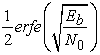(3)

Theoretical BER for BPSK & QPSK in Rayleigh is given by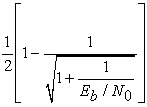(4)

Theoretical BER for 16-QAM in Rayleigh Channel is given by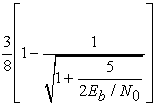(5)

G. OFDM parameters (IEEE 802.11a Standard)

The OFDM physical layer parameters as per IEEE 802.11a Standard are as shown in Table 1 and Table 2.

3. The Simulink Simulation Model

Figure 3 presents the complete block diagram of the OFDM system which was modelled and simulated by us in SIMULINK environment. The main objective of this simulation study is to evaluate the BER performance of the OFDM system using convolutional coding with interleaving. The simulation parameters are obtained from Table 1 and Table 2. A frame based processing is used in this simulation model. The system model was exposed to AWGN channel and Rayleigh fading channel for performance analysis. According to the OFDM standard, information data must be encoded with a convolutional encoder with coding rate R = 1/2, 2/3, or 3/4, corresponding to the desired data rate. The convolutional encoder uses the industry-standard generator Polynomials, [133, 171]. For decoding the convolutional codes the Viterbi algorithm  will be used, which offers best performance according to the maximum likelihood criteria. The input to the Viterbi decoder will be hard-decided bits that are ‘0‘ or ‘1‘, which is referred to as a hard decision. In Soft decision decoding, rather than estimating the coded bit and finding the Hamming distance, the distance between the received symbol and the probable transmitted symbol is found out.Download asVeiw figureFigures index
Figure 3. The SIMULINK model Implemented

Table 2 Physical Layer Parameters [4,5]

Hamming distance, the distance between the received symbol and the probable transmitted symbol is found out.

4. Simulation Results and Discussion

In this section we have presented the simulation results along with the bit error rate (BER) analysis for AWGN channel, Rayleigh fading channel and Rice channel. Three digital modulation techniques including BPSK, QPSK, 16-QAM, 32-QAM, 64-QAM, 128-QAM etc were plotted to see the trade off between system capacity and system robustness. The standard BER of 10-3 was used to determine the minimum performance of the OFDM system for voice transmission. Analysis was done by observing the simulation results.

Figure 4 below shows the spectrum analysis of OFDM system based on 802.11a. By carefully selecting the carrier spacing, the OFDM signal spectrum can be made flat and the orthogonality among the sub channels can be guaranteed.Download asVeiw figureFigures index
Figure 4. The simulated OFDM spectrum

Figure 5 presents the BER plot for BPSK, QPSK, and 16-QAM in AWGN channel. Let us consider a bench mark of BER 10-3. From this we observe that in case of BPSK, QPSK and 16-QAM we are getting a SNR value of around 6dB, 9.8dB and 17.5dB respectively. As BPSK is having the minimum SNR value as compare to QPSK and 16-QAM, with a channel coding gain of 3.8dB w.r.t QPSK and a gain of 11.5dB w.r.t 16-QAM. So it is preferable to use the BPSK modulation technique for transmission of the information data in the AWGN channel.Download asVeiw figureFigures index
Figure 5. BER plot for BPSK, QPSK, 16-QAM in AWGN channel

Figure 6 Given below shows the BER plot for BPSK, QPSK, and 16-QAM in RAYLEIGH channel. Let us consider a bench mark of BER 10-2. From this we observe that incase of BPSK, QPSK and 16-QAM we are getting a SNR value of around 14dB, 19dB and 27.5dB respectively. As BPSK is having the minimum SNR value as compare to QPSK and 16-QAM, with a channel coding gain of 5dB w.r.t QPSK and a gain of 13.5dB w.r.t 16-QAM. So it is preferable to use the BPSK modulation technique for modulation of the information data and transmitting it in the Rayleigh channel.

Now if we compare between the Figure 5 and Figure 6 we get that in both the cases BPSK modulation technique is better as compare to other modulation techniques and we conclude that AWGN channel is better over Rayleigh channel for transmitting of modulated signals. The Figure 7 below shows the plotting between the uncoded BPSK modulated data and convolutional coded modulated data transmitting in AWGN channel. Decoding was done using hard decision decoding as well as with soft decision decoding at the receiver. For a BER of 10-3 we observe that incase of uncoded, cc-(hard) and cc-(soft) we are getting a SNR value of around 6.8dB, 6.7dB and 5.3dB respectively. As the encoded data with soft decoding is having the minimum SNR value, with a channel coding gain of 1.5dB w.r.t. uncoded and a channel coding gain of 1.4dB w.r.t. encoded data with hard decoding. This shows soft decision decoding is better but requires more hardware compared with hard decision decoding.Download asVeiw figureFigures index
Figure 6. BER plot for BPSK, QPSK, 16-QAM in RAYLEIGH channelDownload asVeiw figureFigures index
Figure 7. BPSK (Soft, Hard and Uncoded) in AWGN channel

Next we present in Figure 8 the BER performance of uncoded BPSK modulated data and convolutional coded modulated data transmitting in Rayleigh channel for both hard decision decoding as well as with soft decision decoding at the receiver. For a BER of 10-2 we observe that incase of uncoded, cc-(hard) and cc-(soft) we are getting a SNR value of around 14dB, 4.5dB and 3.5dB respectively. As the encoded data with soft decoding is having the minimum SNR value, with a channel coding gain of 10.5dB w.r.t. uncoded and a channel coding gain of 1dB w.r.t. encoded data with hard decoding. This again shows soft decision decoding is better compared with hard decision decoding.Download asVeiw figureFigures index
Figure 8. BPSK (Soft, Hard and Uncoded) in RAYLEIGH channel

Figure 9 given below shows the effect of using an interleaver in the OFDM system. Here we used 16-QAM modulation technique to interpret the effect. For a BER of 10-3 we observe that the curve of without interleaver is having a SNR value of 17.8dB where as the curve of with interleaver is having a SNR value of 10.2dB. As a result of which we are getting a channel coding gain of 7.6dB. This shows that use of interleaver helps FEC to work properly by converting burst error into single bit error, thereby improvement in BER performance.Download asVeiw figureFigures index
Figure 9. Effect of Interleaving

5. Conclusion

This paper discussed the performance analysis and key concepts of OFDM technology. It was seen that AWGN channel is better over the Rayleigh channel and BPSK is better modulation scheme for low SNR requirement. It is also concluded that convolutional coding and interleaving is mandatory for any wireless transmission. OFDM techniques are quickly becoming a popular method for advanced communication networks. Advances in VLSI technology have made it possible to efficiently implement an FFT block in hardware. This work can be extended by implementation of the complete system in FPGA including the effect of carrier frequency offset and PAPR reduction.

References

  Andreas F. Molisch, “Wireless Communications”, Second Edition, John Wiley & Sons Ltd., 2011.In article  Ramjee Prasad, OFDM for wireless Communications systems‖, Artech House, 2004.In article  Henrik Schulze & Christian Luders, Theory and Applications of OFDM and CDMA‖, John Wiley & Sons, Ltd, 2005.In article  IEEE 802. IF-1993, IEEE Standards for Local and Metropolitan Area Networks: Common Definitions and Procedures for IEEE 802 Management.In article  LAN/MAN Standards Committee of the IEEE Computer Society, Part 11: Wireless LANMedium Access Control (MAC) and Physical Layer (PHY) Specifications-High Speed Physical Layer in the 5 GHz Band, ANSI/IEEE Std 802.11.In article  Agarwal, A., Agarwal, K.: Design and Simulation of COFDM for high speed wireless communication and Performance analysis. IJCA International Journal of Computer Applications 2, 22-28 (2011).In article  Agarwal, A., Patra, S.K.: Performance prediction of Eureka-147 DAB system Using Interleaving and different Coding rates. In: Proceedings of the IEEE International Conference on MEMS, Nano & Smart Systems, ICMENS, vol. 403-408, pp. 4119-4125 (2011).In article View Article  John. G. Proakis, "Digital Communications", 3rd edition, McGraw-Hill, 1995.In article  Akram Ramezani, “A novel technique for time synchronisation in OFDM systems,” Journal of Telecommunications, vol 10, issue 2, September 2011, pp.18-21.In article